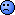## FDA's RSABE on NTIDs in R [RSABE / ABEL]

Dear CECIF,

❝ I need some…clarifying, if you will.

I will try my best.

❝ I am also, trying to replicate the FDA guidance on warfarin. I am using R. However I am stuck in steps 2 and 3.

❝ In step 2 I am able to calculate ((YR-YT)^2)-theta*swR2, but it says I should find the upper 95% CI using Howe´s method. Sincerely I just read Howe's method (from the reference in the FDA guidance) for like 4 hours and still can't understand how to use it (maybe it´s because I am not a certified statistician). Can somebody give me a step by step on how to do this?.

Since you are working with R have a look at this thread, especially at this post.
See here and especially here for some discussions about how to calculate the scaled ABE criterion (linearized) including it's upper 95% CI according to Hyslop's method, sometimes the name Howe is also used.

❝ An additional comment on step 2: once I find the upper 95% CI, What I am supposed do to with it? it does not seem to appear on the subsequent steps (or at least not alone). I just saw your presentation on RSABEs, and you use the criteria "Bioequivalent If 95% upper CI <0" where does this criterion comes from?

This is simple the BE decision to be taken based on the upper 95% CI of the linearized RSABE criterion. Read papers on HVD to understand where it comes from.

❝ In step 3 “every study should pass the scaled and unscaled and scaled bioequivalence limits”: “every study" refers to:

❝ 1. Period 1 vs period 2 (regular bioequivalence study)

❝ 2. Period 3 vs period 4 (replicated study)

❝ 3. Pooled analysis (Average periods 1 and 3 vs average period 2 and 4)

❝ 4. Repeated crossover analysis considering all four periods

❝ Did I miss any?

No, you don't miss. But your understanding is a miss-understanding.
The sentence means: The study under consideration must pass the following criteria:
• Scaled ABE test: 95% CI of RSABE criterion <0
• Conventional ABE test: 90% CIs within acceptance range
• Upper 90% CI of the ratio of intra-subject standard deviations of Test and Reference <= 2.5

❝ In the study 4 mentioned above is it correct to use the same methods for calculation of BE limits as the others, but adding another factor for the variable of “replication” in the ANOVA?

But in the Warfarine guidance a mixed model analysis (SAS Proc MIXED code) is recommended.
Be aware, this is not implementable in R one to one.
What you can do is: Use the 90% CI you already has calculated during calculation of the 90% CI of the RSABE criterion. I myselve never understood why the FDA uses two different methods, one for calculating the RSABE criterion and another one for calculating the conventional 90% CIs.

❝ And finally, is it necessary to comply with all four steps (and multiple criteria) of this guidance to demonstrate bioequivalence? ...

Yes.

Regards,

DetlewIng. Helmut Schütz